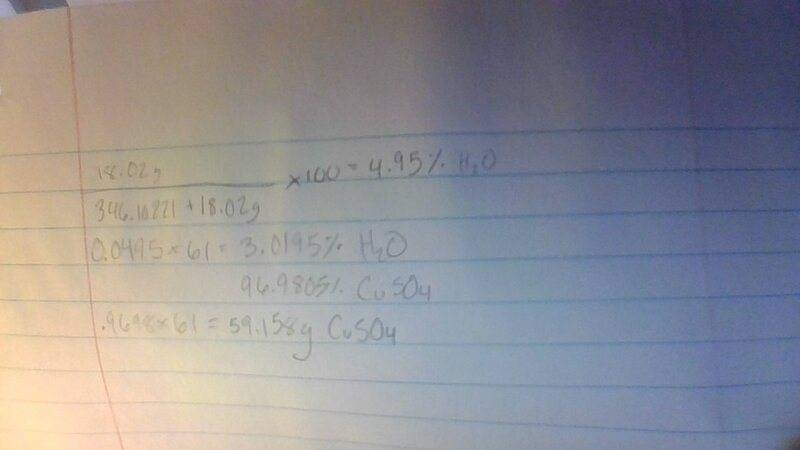# Mass of an anhydrous compound

• Chemistry
Homework Statement:
What is the mass of CuSO4 if the mass of CuSO4.H2O is 61 grams
Relevant Equations:
CuSO4.H2OLast edited:

Homework Helper
Gold Member
Have you read the rules for the forum?

•Astronuc
Have you read the rules for the forum?
There are rules?

Homework Helper
Gold Member
You need to make an attempt at the solution, show some work.

•Astronuc
I just tried re working it and showing work but I have no idea what I'm doing and I tried using my noted but its no help

Homework Helper
Gold Member

•Astronuc
on paper?

Staff Emeritus
Gold Member
Post it here so someone can help you figure out what you're doing wrong.

•AstronucMentor
In the future please show your effort when starting the thread, not an hour after. We can't comment on things we don't see.

Your logic is mostly OK, but check the molar masses you are using.

•Astronuc and Bystander
Homework Helper
Gold Member
Homework Statement:What is the mass of CuSO4 if the mass of CuSO4.H2O is 61 grams

Relevant Equations:CuSO4.H2O
Calculate or find the formula weights for cupric sulfate and cupric sulfate tetra-hydrate mono-hydrate. You can find the fraction of cupric sulfate in the hydrated compound. You will be able to form a very straightforward proportion.

Last edited:
Homework Helper
The question refers to the monohydrate.

Homework Helper
Gold Member
Calculate or find the formula weights for cupric sulfate and cupric sulfate tetra-hydrate. You can find the fraction of cupric sulfate in the hydrated compound.
"Penta-hydrate," I'm unaware of the existence of either mono-, or tetra-hydrates. Did not point that fact out, since it provides/exemplifies additional instructional/nomenclatural/pedagogical opportunities for the OP. The significance of small whole numbers/fractions in discussing "hydrates" is important.

Homework Helper
•